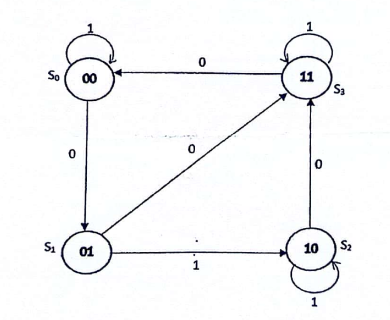### Exam Year

Tribhuvan University

Institute of Science and Technology

2079

Bachelor Level / first-semester / Science

Computer Science and Information Technology( CSC111 )

Digital Logic

Full Marks: 60 + 20 + 20

Pass Marks: 24 + 8 + 8

Time: 3 Hours

Candidates are required to give their answers in their own words as far as practicable.

The figures in the margin indicate full marks.

Section A

Attempt any Two questions: ( 2*10=20)

1

Design the essential circuit with respect to the following state diagram using T flip flops.2

Implement F= ∑ (0,1,4,5,7) using :

a) Multiplexer

b)Decoder

c)PLA

3

Differentiate between combinational circuit and sequential circuit. Design mod-10 synchronous counter using J-K flip flop. Show necessary truth tables and k-maps.

Section B

Attempt any Eight Questions ( 8*5=40)

4

Provide one example where shift left operation can be used. Explain serial-in-serial-out register.

5

Carry out the following tasks :

a) Perform 2’s complement subtraction of 010110-100101

b)Represent decimal number 10 in it’s BCD form

6

Derive the Boolean expression for sum and carry of half adder. Draw it combinational circuit. Implement it using only NOR gates.

7

Express F’ = (x + y z )’ as a sum of min-terms.

8

Minimize the following Boolean function using K-map :

F(A,B,C,D)=∑m (0,1,2,5,7,8,9,10,13,15)

9

Write the practical implications of down counter ? Explain BCD ripple counter

10

Design a combinational circuit with three inputs and one output. The output is 1 when the binary value of the inputs is an even number.

11

Differentiate between ROM and PLA. Explain various types of ROM.

12

Write short notes on (Any two)

a) Positive Logic

b) I2L

c) ASCII